#### Physical methods of structural Investigation, Chemistry tutorial

Introduction:

In simple language, physical techniques of structural investigation are methods of acquiring information regarding a newly synthesized complex. Much of what we know nowadays regarding properties of complexes comes from the physical methods of structural investigation. Working at the atomic and molecular level, physical processes of structural investigation comprises the application of an array of physical processes that can provide information on the complex under investigation. As there are many techniques, it is significant to know the techniques most appropriate for getting the information desired. Once a new complex is synthesized and purified, the primary investigation is to know elements that comprise the complex which associates to the chemical composition. The next investigation must be on the shape of the complex, which a far more complex comprises defining what components are comprised and how they are assembled altogether.

Synthesis and purification of the complexes may be difficult; the techniques involved in the structural investigation are more complex and multi-tasking. Defining molecular structure in both solution and the solid state needs a broad range of physical techniques for characterization.

Quite a number of physical techniques have been used to study the properties of metal complexes in order to give information on molecular structure and reactivity. The characterization in metal complexes comprises isolating a complex in the solid state. This method is difficult and might be complex if there exist differences in the nature of the complex in solution and the solid state. The physical methods used in the characterization of complexes comprise; electronic absorption spectroscopy, magnetic measurement, vibrational spectroscopy, conductivity, elemental analysis, thermal analysis, mass spectroscopy, x-ray crystallography, nuclear magnetic resonance, electron spin resonance and others. The list is in reality endless as various methods are employed for various purposes of investigating properties of complexes. It is thus significant to gain knowledge on some of such investigative techniques.

Methods used in structural investigation of complexes:

The comprehensive structural investigation of complexes comprises most of the analyses providing various types of information on nature and structure of complexes. It is thus significant to know what information can be obtained from each analysis taken out on complexes.

Elemental analysis of a pure complex will give information on the percentage composition of the different elements (C, H, N and S) which comprise the complex. This will help to predict an appropriate formula for the complex. Thermal analysis might be taken out to find out change in mass by temperature. This assists to know the number of waters of crystallization present in the complex and as well assists to find out the heat stability of the complex.

Conductivity measurement assists to differentiate between ionic and neutral complexes. Magnetic properties of the complexes are obtained via magnetic measurement. Electronic (UV-VIS) spectroscopy gives information on the geometry of complexes and vibrational spectroscopy assists to find out the possible binding site(s) of ligand to complex. The molecular mass of a complex can be acquired by mass spectroscopy. Whenever an appropriate crystal of a complex is available, x-ray crystallography can be employed to establish the structure of the complex. The other forms of analyses are as well carried out to get more information on other properties of complexes.

This is not enough to know the different methods employed in structural investigation of complexes, it is very significant to get acquainted to the principles behind some of such analyses to facilitate interpretation and understanding of results acquired from them. This course shall concentrate on electronic spectroscopy, vibrational spectroscopy and magnetic measurement.

Electronic Spectroscopy:

One of the notable features of the transition metal ions and their complexes is their colorful appearances. This is attributed to the partial filling of the d-orbitals that makes them capable of absorbing the light energy in the visible region leading to electronic transitions between various energy levels in split d-orbitals. In an electronic transition, an electron is excited from an occupied to an empty orbital. The energy of these transitions generally corresponds to light in the near IR, visible or UV region of the electromagnetic spectrum. In d-block complexes different kinds of electronic transitions are possible based on the nature of their constituents. Some of such transitions take place within the ligand (that is, intraligand transition), in the split d-orbitals (that is, ligand field or d-d transition) and/or between the metal d-orbitals and ligand orbitals (that is, charge transfer transition).

To provide description on the colours of complexes, it is essential to have understanding of the complementary colours. Whenever light of a specific colour is absorbed through a complex, the complementary colour to the absorbed colour is observed. For illustration, whenever white light (that is, having a broad spectrum of all visible wavelengths) is allowed to pass via a substance that consists of capacity to absorb blue light, the substance will appear in orange since the complementary colour of blue is orange. A simple manner to remember the complementary colour is shown below by employing colour wheel.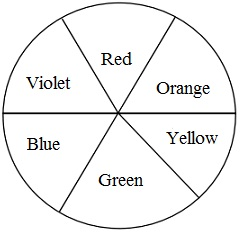Fig: Colour wheel

Whenever light intensity 'Io' is allowed to pass via a standard solution of a complex in a UV/Visible spectrophotometer, electron(s) in the complex will absorb part of the light and be promoted to the higher energy level whereas the unabsorbed light with intensity 'I' will be transmitted via the solution.  A graph of log [Io/I] (Absorbance) can be made against wavelength in nm. This plot is termed as the electronic absorption spectrum from which the different absorptions of the compound can be found out. The intensity of the absorption (that is, molar absorptivity (ε)) can as well be determined by using Beer-Lambert Law, mathematically represented as;  ε = A/lc, A = absorbance,  l = path length (cm) via the solution and c = concentration in mol/ dm3. The unit of ε is L/mol cm. This is possible to compute wave number (ν) in cm-1 equivalent to the wave length (λ) by using the expression:  ν = 1/ λ. With high 'ε', complexes are intensely colored and appear extremely bright.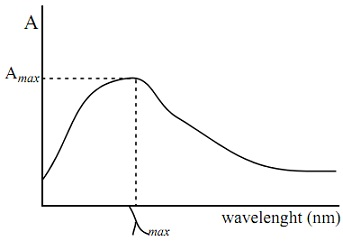Fig: Example of Electronic Absorption Spectrum

Transition in Complexes:

Transitions in the complexes can be grouped into three groups; intraligand transitions, ligand field transitions and charge transfer transitions.

=> Intraligand Transitions:

These are the transitions which depend on the nature of chromophores (that is, group of atoms capable of absorbing light energy) present in the ligands. Such chromophores comprise organic functional groups like alkenes, aromatic hydrocarbons, heterocyclic compounds, amines, alcohols and ethers.

n → σ* transition: In saturated molecules that comprising heteroatoms having non-bonding lone pairs of electrons, the transition of the lowest energy will be n → σ*. This is possible as both the bonding and nonbonding orbitals are filled by electron and the highest occupied molecular orbital (HOMO) is the non-bonding orbital n whereas the lowest unoccupied molecular orbital (LUMO) is antibonding σ*. Illustrations of ligands with this transition are: amines, alcohols, ammonia, water and ethers.

n → π* transition: This is possible in ligands having both nonbonding and π bonding molecular orbitals. In such a ligand, the HOMO will be n, LUMO will be π* and transition with the lowest energy will be n → π*. Illustrations of such ligands are ketones (R-CO-R), aldehydes (R-CHO), esters (RCOOR), amides (RCONH2) and carboxylic acids (RCOOH).

π →π* transition: The ligands having double double bonds or triple bonds devoid of any heteroatom will have transition of lowest possible energy to be  π →π*. Illustrations are olefins, conjugated dienes and the aromatic hydrocarbons.

Unsaturated heterocyclic compounds (like 1, 10-phenanthroline, 2, 2'-bipyridine and pyridine, multiple transitions) have various transitions comprising; π → π*, n → π* and n → σ*. The ligand transitions are frequently in the ultra violet range of the spectrum however with extended conjugations, a few transitions in the visible regions will be inevitable.

Charge transfer Transitions:

The electron can be excited from a metal orbital to ligand orbital in the transition complex. This is made possible whenever, the metal consists of electron in t2g orbitals (that are nonbonding orbitals) and the ligand has appropriate empty orbitals (π*). The transition of electron in this method will lead to decrease in electron density around the metal centre (that is, oxidation). This transition is termed as Metal to Ligand Charge Transfer (MLCT) transition generally found in the complexes with metal ion in low oxidation state, which can be simply oxidized. Illustrations are complexes of Ti3+ and Cu+. Usually, Charge Transfer (CT) transitions are more intense and might likely mask d-d transitions however they take place at higher wave number generally above 40, 000cm-1 with molar absorptivity above 10, 000 L mol-1 cm-1. This is because the CT transitions are spin-allowed and Laporte-allowed. Though, d-d transitions are just spin-allowed.

What can be tagged the reverse of MLCT is Ligand to the Metal Charge Transfer (LMCT) where electron density is transferred to the metal, which is thus reduced in the excited state. The higher the oxidation states of the metal concerned, the simpler these reductions will be, and therefore the lower the LMCT energy. LMCT transitions in the visible region of the spectrum give intense colour, as is found with permanganate (MnO4-) a d0 complex, in which no d-d transition is expected. The other illustrations comprise: TiF62-, CrO42- and TcO4-

In a complex having two metal ions in different oxidation state, there might be transfer of electron from orbital of metal in the lower oxidation state to that in higher oxidation state. This transition is termed as Metal to Metal Charge Transfer and it can be noticed in KFe[Fe(SCN)6] and [[AuIIICl4][AuICl2]]2-.

Ligand field Spectra (d-d transition):

The Ligand field spectra results from the transition in metal d-orbitals which have been split because of the ligand field on coordination. They are as well termed as d-d transitions as they take place in d-orbitals. For proper understanding of d-d transitions, knowledge of interactions between electrons in the d-orbitals is highly crucial. The electron in a particular atom has a set of four quantum numbers; the principal (n), the subsidiary (l), the magnetic (m) and the spin quantum numbers. Interaction can take place between spin angular momentum and orbital angular momentum of the single electron. Though, if two or more unpaired electrons are present with an atom, three different interactions are possible between the angular momenta; spin-spin, orbit-orbit and spin-orbit interactions. The angular momenta are vector quantities which interact (couple) in accord by quantum-mechanical rules. In spin-spin coupling schemes, the spin angular momenta of the electrons couple to provide an overall resultant spin (S). Likewise, the orbital angular momenta of the electrons couple to provide an overall resulting orbital angular momentum (L). The resultant vector quantities (S and L) couple to provide the total angular momentum vector (J) for the atom.

Coupling take place by this scheme, which is termed as L-S or Russell-Saunders coupling? The coupling scheme can be deduced as;

S = ∑si =  s1 +  s2,  s1 +  s2 - 1, ...., s1- s2, here 'S' stands for vector sum of the resulting spin angular momenta and si symbolizes the spin of the individual electron.

L = ∑li =  l1 +l2,  l1 +  l2 - 1, ....,  l1 - l2, here 'L' stands for vector sum of the resulting orbital angular momenta and li symbolizes the orbital angular momentum of the individual electron.

J = L + S = L +S, L + S - 1... L-S, here 'J' stands for vector sum of the resulting orbital and spin angular momenta.

In Russell-Saunders coupling, the spin-spin coupling is supposed to be greater than orbit-orbit coupling which is as well greater spin-orbit coupling for the first row transition metals.

Illustrations: for d1 electronic configuration, s = +1/2 and l = 2, since only one electron is comprised only spin-orbit coupling is applicable. J = 2 + 1/2, 2 - 1/2. J = 5/2 and 3/2.

It will be noted that spin of electron is either -1/2 or +1/2 and atomic orbitals designated as s, p, d, and f have values 0, 1, 2, 3 and 4 correspondingly.

In a manner identical to that through which the atomic orbitals are designated as s, p, d, or f, the letters S, P, D and F correspond to the values of 0, 1, 2, and 3, correspondingly, for the resulting angular momentum vector (L). After the values of the vectors L, S, and J have been found out, the overall angular momentum is illustrated by a symbol termed as a term symbol or spectroscopic state. This symbol is constructed as (2S + 1)LJ where the quantity (2S + 1) is termed as the multiplicity. For one unpaired electron system s = +1/2, (2(1/2) + 1) = 2, and a multiplicity of 2 signifies doublet state. Thus for one unpaired electron system the term the given are obtained S = 1/2, L = 2 and J= 5/2 and 3/2, which provide the term symbols 2D5/2 and 2D3/2

The spectroscopic terms for d1 and d9 are similar as both have the similar number of unpaired electron. The other related d-configurations having the similar term symbols can be found out by utilizing the expression dn = d10-n.

For d2 and d8 configurations, the term symbols can be likewise determined. These are two electron systems ↑ ↑ _ _ _ with s1 = + 1/2, s2 = +1/2, l1 = 2 and l2 = 2

S = s1 + s2, s1 + s2 - 1... s1 - s2 = 1 and 0

L = l1 + l2, l1 + l2 - 1... l1 - l2 = 4, 3, 2, 1 and 0

J = L + S = L + S, L + S - 1.... L-S, with L = 4 and S = 1, J = 5, 4, 3 multiplicity is 2(1) + 1= 3

Term symbols are 3G5, 3G4 and 3G3. The other symbols are derived in an identical manner;

With L = 3 and S = 1, J = 4, 3, 2 Term symbols are 3F4, 3F3 and 3F2

With L = 2 and S = 1, J = 3, 2, 1 Term symbols are 3D3, 3D2 and 3D1

With L = 1 and S = 1, J = 2, 1, 0 Term symbols are 3P2, 3P1 and 3P0

With L = 4 and S = 0, J = 4 Term symbols are 1G4 Note 2(0) + 1 = 1 singlet state

With L = 3 and S = 0, J = 3 Term symbols are 1F3

With L = 2 and S = 0, J = 2 Term symbols are 1D2

With L = 1 and S = 0, J = 1 Term symbols are 1P1

With L = 0 and S = 0, J = 0 Term symbols are 1S0

NOTE: L; 0, 1, 2, 3, 4, 5 and 6 encompass symbols S, P, D, F, G, H and I

Not all the derived terms are permitted by Pauling exclusion principle, which states that no two electrons should have the similar values for the four quantum numbers in a particular atom and Hund's rule which states that degenerate states should be singly filled before pairing.

To find out the allowed term symbols, the microstates of the electronic configuration should be produced and then arranged to get the derived term symbols. This is an extremely complex however interesting procedure that should be carefully studied in order to encompass indebt knowledge of electronic transition. The microstate for d1 and d9 is illustrated below:

S = 1/2, MS = S, S -1....0...-S, Ms = +1/2, -1/2. Ms is possible spin microstate

L = 2, ML = L, L-1....0.....-L, ML = 2, 1, 0, -1, -2.  ML is possible orbital microstate.

From various spin and orbital microstates possible, a table of all the combined microstates can be produced. The microstates are the possible orientations of the electron that are permitted by the Pauling's and Hund's rules. A microstate is symbolized with the orbital in which the electron is placed with superscript symbolizing the spin of the electron. The total number (N) of microstates for a particular d-configuration can be computed from the expression;

N = [(2(2l+1)!]/[x!(2(2l+1)-x)!] Here 'l' is electronic orbital angular momentum and 'x' is the number of unpaired electrons.

For d1 configuration, l = 2, x = 1 and N = [2(2(2)+1)!]/[(1!(2(2(2)+1)-1)!] = 10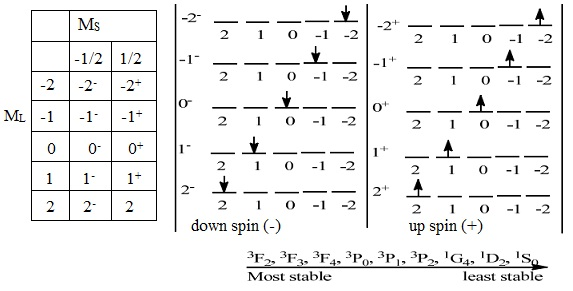Fig: Ligand field spectra

From the microstates, just 2D term is possible as illustrated by the colored microstates. For d2 and d8 the highest L = 4, ML = 4, 3, 2, 1, 0, -1, -2, -3, -4. The highest S = 1, MS = 1, 0, -1

N = (2(2(2)+1))!/2!(2(2(2)+1)-2)! = 45, note that l = 2 and x = 2 for d2 or d8.

Splitting of free ion terms in octahedral/tetrahedral fields:

Just as the free ions split under the effect of external magnetic fields, the approach of the ligands as well splits the free ion terms. The splitting pattern based on the geometry of the complex. The spectroscopic states are split to components which encompass the similar multiplicity as the free ion states from which they occur. A single electron in a d orbital (d1 and d9) gives rise to a 2D term for the free ion (that is, uncoordinated), however in an octahedral field the electron will reside in a t2g orbital, and the spectroscopic state for the t2g1 configuration is 2T2g. If the electron were excited to an eg orbital, the spectroscopic state would be 2Eg. Therefore, transitions between 2T2g and 2Eg states would not be spin forbidden as both states are doublets.

It will be noted that lowercase letters are employed to illustrate orbitals, while capital letters illustrate spectroscopic states.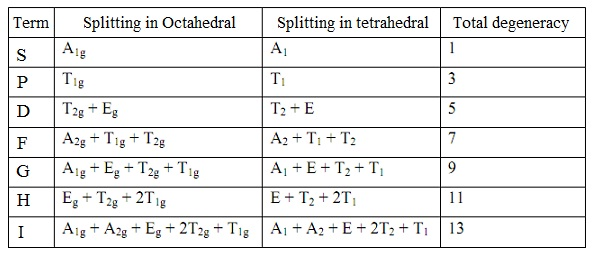Fig: Splitting of free ion terms

Selection rule:

Spin Rule: It states that transitions between states of the similar multiplicity are allowed however transitions between the states of different multiplicities are not allowed. Illustrations: transitions between 2Tg and 2Eg, 3T1g and 3T2g are allowed however transitions between 2Tg and 1Eg, 4T1g and 3T2g are not allowed or forbidden.

Larporte Rule: It states that transitions between state having ΔL = ±1 are allowed however those with change in L greater or less than ±1 are forbidden. This rule signifies change in parity or symmetry of orbital; g → g and u → u are allowed however g → u and u → g are not allowed. S → s, p → p, s → d and p → f are not allowed however s → p, p → d and d → f are allowed.

Molar Absorptivity (εmax):

The molar absorptivity finds out the intensity of a particular absorption, a band having high εmax will be intensely colored whereas those having low εmax will not. The value of εmax based on the transition. Transitions which are Spin and Larpote allowed encompass high εmax.

Transition                                              εmax (L mol-1cm-1)       Examples

Spin-forbidden (and Laporte forbidden)              < 1                   [Mn(H2O)6]2+,d5

Laporte-forbidden (spin allowed)                     20-100                [Ti(H2O)6]3+,d1

Laporte-allowed                                                 ~ 500                [NiCl4]2-, d8

Symmetry allowed (charge transfer)            1000-50000             [MnO4]-, d0

Transitions in Octahedral field:

=> d1 and d9 complexes:

d1 complex illustration is [Ti(H2O)6]3+ and d9 complex example is [Cu(H2O)6]2+, ground term is found out and the split terms occurring from the ground term are arranged based on stability (that is, the number of possible arrangements in ground state).

Ground term is 2D for d1and d9

2D splits into 2Eg and 2T2g for d1 and d9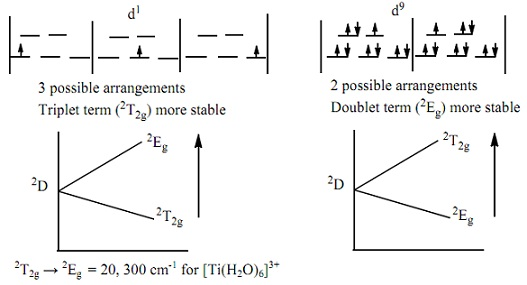Fig: d1 and d9 complexes

=> d2 and d8 complexes:

d2 complex example is [V(H2O)6]3+ and d8 complex illustration is [Ni(H2O)6]2+. Ground term is 3F for d2 and d8 with 3P excited state of similar multiplicity with ground state.

3F splits to 3A2g, 3T2g and 2T1g while 3P gives rise to 3T1g.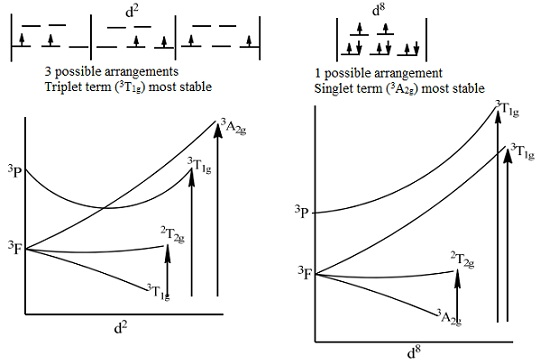Fig: d2 and d8 complexes

From the splitting pattern in octahedral field, three transitions are possible in d2 and d8 complexes. Such transitions are noticed at particular bands for individual complexes. In the spectrum of [V(H2O)6]3+; 3T1g 3T2g takes place at 17,800cm-1 and 3T1g3T1g (P) takes place at 25,700cm-1. The third transition 3T1g3A2g is weak although of higher energy. In [Ni(H2O)6]2+, the bands are 8500 cm-1 for 3A2g3T2g  13, 800 cm-1 for 3A2g3T1g (F)  and 25, 300 cm-1 for 3A2g3T1g (P).  Appropriate diagrams in which all different splitting pattern are well described is established by Orgel.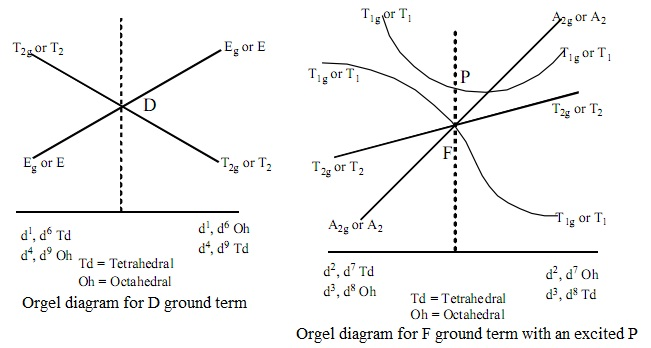Fig: Orgel Diagram

Tanabe-Sugano Diagrams:

Orgel diagrams lets a qualitative illustration of the spinal-allowed electronic transitions expected for states derived from D and F ground terms whereas Tanabe-Sugano (TS) diagrams provide a semi-quantitative approach, comprising both high and low spin ions. TS diagrams cover both the spin-allowed transitions and the spin-forbidden transitions which are weak and might not probable be noticed. TS diagrams are complex in nature however a good understanding of the diagrams is very helpful in spectra interpretation. TS diagrams are plots of ratio of energy (E) of transition to Racah parameter B, (E/B), against ratio of Δ0/B. Various diagrams are available for different d configurations. The TS diagram d2 is illustrated in the diagram below.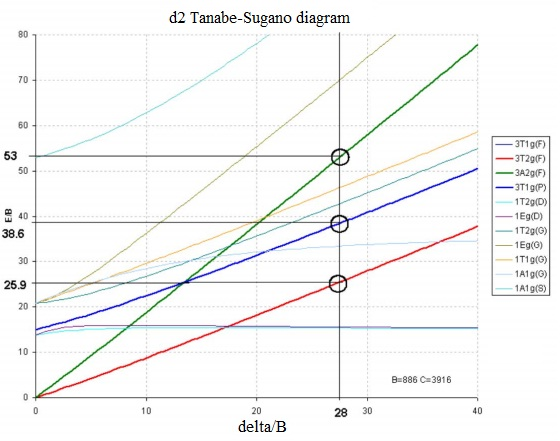Fig: Tanabe-Sugano Diagrams

Transitions in Tetrahedral Field:

Usually, tetrahedral complexes encompass more intense absorptions as compare to octahedral complexes. This is as an outcome of the absence of a centre of symmetry in tetrahedral complexes that makes transitions between d orbitals more intense as compare to in octahedral complexes. From the Orgel diagrams, the d orbitals for tetrahedral complexes are split in the opposite fashion to the octahedral complexes.

Given any dn configuration the ground term can be simply found out by given such steps.

1) Filling the electrons to the 5 degenerate d-orbitals and compute the |L| value for the ground term based on the positions of the electrons in the orbitals. The filling should follow Hund's rule and Pauling Exclusion Principle.

2) The multiplicity is acquired as number of unpaired electrons plus one (n + 1)

Vibrational Spectroscopy:

Similar to the bonds in functional groups of organic compounds, bonds inorganic compounds produce infrared spectra which can be employed in their characterization. Dissimilar to the bonds in functional groups of organic compounds, the infrared bands for inorganic materials are wider, fewer in number and appear at lower wave numbers as compare to those observed for the organic materials. Whenever an inorganic compound forms covalent bonds in an ion, it can provide a characteristic infrared spectrum. The bands in the spectra of ionic or coordination compounds based on the structures and orientations of the central metal and the ligands. Usually, complexes having organic ligands will encompass characteristic infrared absorption bands of the functional groups in the ligands. However as often expected such bands are expected to shift on co-ordation particularly bands which are characteristics of the binding site in the organic ligands. The fundamental principle of infrared is studied major in organic chemistry however it will be of importance to mention significant features of this principle as it will give information on why bonds absorb infrared electromagnetic radiation.

Infrared spectroscopy is a significant analytical methods employed to study the nature of bonding in a particular chemical compound to find out the different functional groups present. This is made possible as various bonds have different and unique region of infrared absorption. Infrared spectroscopy is one of the best analytical instruments as virtually all forms of samples comprising in organic samples can be simply studied on it. Infrared spectroscopy is based on the vibrations of the bonds joining atoms altogether in a molecule.

The product of infrared analysis is the infrared spectrum that is obtained via passing infrared radiation via a sample and finding out what fraction of the incident radiation is absorbed at a specific energy just similar to in electronic spectroscopy. The peaks in the spectrum are features of the frequencies of absorption of bonds present in the sample analyzed. The spectrum is a plot of percentage transmittance against wave number deduced in cm-1. By the wave-number scale, infrared absorptions for all organic compounds (that is, functional groups) take place from 4000 cm-1 to 400 cm-1. Though, those of ionic compounds such as NaCl and KBr have absorption bands at higher wave-number. In complexes, the bonds between the metal and the binding site in an organic ligand absorb at lower wave-number.

Only compounds having dipole moment are capable of absorbing infrared energy and are stated to be infrared active whereas molecules without dipole moment are stated to be infrared inactive such group of compound are studied by using Raman Spectroscopy. Therefore molecules such as water, ammonia are active however molecules such as O2, Cl2 are inactive to infrared. The dipole moment of an infrared-active molecule changes as the bond expands and contracts. Via comparison, an infrared inactive molecule will encompass its dipole moment remains zero no matter how long the bond vibrates.

The basic modes of vibration in compounds are stretching and bending. The stretching can be symmetric or asymmetric whereas the bending can be wagging, twisting, rocking or deformation. Such modes have different regions of infrared absorption. The energy of stretching mode is generally higher as compare to that of bending mode.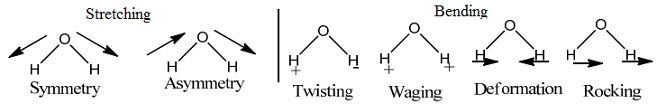Fig: Fundamental modes of vibration in compounds

Interpretation of Infrared spectra:

After the infrared spectrum has been obtained, it should be interpreted to find out the functional groups present in the sample. Spectrum is not complex such as electronic spectrum in ultra violet/visible spectroscopy. The different bands in infrared in spectrum have been grouped to what is termed as group frequencies. The characteristic group frequencies are far infrared (that is, less than 400cm-1), mid-infrared (400 to 4000cm-1) and near infrared (4000 to 13,000cm-1) regions.

=> Far-Infrared Region:

The far-infrared region, by definition, comprises the region between 400 and 100 cm-1. This region is more limited as compare to the mid infrared for spectra-structure characterization. Though, it does give information regarding the vibrations of the molecules of heavy atoms. Intramolecular stretching modes comprising heavy atoms can be useful for characterizing compounds having halogen atoms, organometallic compounds and inorganic compounds.

=> Near-Infrared Region:

The absorptions in the near-infrared region (13000 - 4000 cm-1) are generally overtones or combinations of the basic stretching bands that take place in part of the mid infrared region (3000-1700 cm-1). The bands involved are generally because of C-H, N-H or O-H stretching. The resultant bands in the near infrared are often weak in intensity and the intensity usually reduces by a factor of 10 between the adjacent successive overtones. The bands in the near infrared are frequently overlapped, making them less helpful as compare to the mid-infrared region for qualitative analysis.

=> Mid-Infrared Region:

The mid-infrared spectrum (4000-400 cm-1) is generally grouped into four regions and the character of a group frequency can be found out by the region in which it is localized. The regions are: the region of absorption for bond between hydrogen and heteroatom (O-H, C-H, N-H) stretching region (4000-2500 cm-1), the triple-bonds (C≡N, C≡C) region (2500-2000 cm-1), the double-bond (C=C, C=N, C=O) region (2000-1500 cm-1) and the fingerprint (characteristic of the molecule being analyzed) region (1500-600 cm-1). For the fundamental vibrations in the 4000-2500 cm-1 region, O-H stretching provides broad band that takes place in the range 3700-3600 cm-1. N-H stretching is mainly found in the narrow range 3400-3300 cm-1 by the sharper absorption as compare to O-H stretching and can be simply differentiated, with NH2, resonance take place and two close sharp bands are seen rather than one. C-H stretching bands takes place at the range 3000-2850 cm-1 in most of the aliphatic compounds. Whenever the C-H bond is next to a double bond or aromatic ring, the C-H stretching band takes place between 3100 and 3000 cm-1. Triple-bond stretching absorptions take place in the 2500-2000 cm-1 region because of the high force constants of bonds. C≡C bonds absorb between 2300 and 2050 cm-1, whereas the nitrile group (C≡N) takes place between 2300 and 2200 cm-1. Such groups can be simply recognized as C≡C stretching is often very weak, whereas C≡N stretching is of medium intensity. These are the most general absorptions in this region; however you might come across various X-H stretching absorptions, where X is a more massive atom like phosphorus or silicon. Such absorptions generally take place near 2400 and 2200 cm-1, correspondingly.

The main bands in the 2000-1500 cm-1 region are caused due to C=C and C=O stretching. Carbonyl stretching is an extremely easy absorption to recognize in this region. This is generally the most intense band in the spectrum. The nature of C=O bond can as well be confirmed as there are regions for different carbonyl contain organic moieties. It is very germane to know that metal carbonyls might absorb above 2000 cm-1 C=C stretching is much weaker and takes place at around 1650 cm-1, however this band is often not noticed for the symmetry or dipole moment reasons. C=N stretching as well takes place in this region and is found to be sharper and more intense. The bands in the region 1500-650 cm-1 are characteristic of the molecule be analyzed therefore region is termed as the fingerprint region. It is very significant to note that most of the bands might differ from one compound to the other and as well from one environment to the other. This signifies that the values are not fixed and in few cases variation might takes place at 100cm-1.

Magnetic measurement:

Movement of the electrical charge produces magnetic field in any material. The magnetism is thus a characteristic property of all the materials which have electrically charged particles that are in continuous motion and for most purposes can be considered to be totally of electronic origin. In an atom, the magnetic field is because of the coupled spin and orbital magnetic moments related by the motion of electrons. The spin magnetic moment is because of the precession of the electrons regarding their own axes while the orbital magnetic moment is because of the motion of electrons around the nucleus. The resulting combination of the spin and orbital magnetic moments of the constituent atoms of a material gives rise to the observed magnetic properties. Most of the transition metal compounds are paramagnetic because of the presence of unpaired electrons. Magnetic moment of each and every such electron is related by its spin angular momentum and orbital angular momentum.

Principle of Magnetism:

According to the Lenz's Law, which defines that whenever a substance is put in a magnetic field, H, the field in the substance, B, varies from H by the induced field, which is proportional to the intensity of magnetization (I).

This can be mathematically represented as B = H + 4πI, here 'B' is the magnetic field in the substance, 'H' is the applied magnetic field and 'I' is the intensity of magnetization. By dividing via with H, It can as well be represented as:

B/H = 1 + 4πI/H, or P = 1 + 4πK

Here B/H is known as the magnetic permeability (P) of the material and 'K' is the magnetic susceptibility per unit volume, (I/H). By definition, K in a vacuum is zero, here B = H. It will be noted that P = B/H = 1

This is generally more convenient to compute mass as compare to volume and the mass susceptibility, χg is related to the volume susceptibility, K, via the density

χg = K/d here 'd' is the density

It is essential to compute this quantity on the basis that can be associated to atomic properties, it thus transformed to molar susceptibility by multiplying the mass susceptibility with relative molar mass (RMM) of the atom.

χm = χg * RMM

All the materials are known to encompass inherent diamagnetism; it is significant to comprise a term which will reflect this diamagnetism of the material being measured to encompass a corrected molar susceptibility.

χcorr = χm + χdia

Curies Law is followed through Normal paramagnetic substances

χm = C/T

Here 'C' is the Curie constant. A plot of 1/Χ versus T must give a straight line by the slope 1/C passing via the origin (0 K). Some of the substances provide a straight line that intercepts just a little above or below 0K and these are stated to obey the Curie-Weiss Law:

χ = C/(T ± Φ) here 'Φ' is termed as the Weiss constant.

The constant C is provided by the Langevin expression that relates the susceptibility to the magnetic moment;

m = Nμ2/3 kT

Here 'N' is Avogadro's number; 'k' is the Boltzmann constant and 'T' be absolute temperature.

μ = 2.828 √(χm.t)

Based on the quantum mechanics, the magnetic moment is based on both spin and orbital angular momentum contributions. The spin-only (s.o.) formula is represented as:

μs.o. = √4S(S+1) or μs.o. = √n(n+2)

Here 'S' is the resulting spin angular momentum and 'n' is the number of unpaired electrons. This expression can be modified to comprise orbital contribution to the magnetic moment.

μ = √(4S(S+1) + L(L+1)

Types of Magnetic Behaviors:

Diamagnetism:

Diamagnetism is the phenomenon in several materials in which the susceptibility is negative (χm) that is, the induced magnetism opposed the applied field. It occurs from the precession of spinning charges in a magnetic field. The magnetization is in the opposite direction to that of the applied field, that is, the magnetic susceptibility is negative (χm < 0). However all the substances are diamagnetic, it is a weak form of magnetism and might be masked by other, stronger forms. Diamagnetism yields from changes induced in the orbits of electrons in the atoms of a substance by the applied field, the direction of change (that is, in accordance with Lenz's law) opposing the applied magnetic flux. There is therefore a weak negative susceptibility (χm < 0)

Diamagnetism is an extremely weak form of magnetism which is only exhibited in the presence of an external magnetic field. This is the result of changes in the orbital motion of electrons because of the external magnetic field. The induced magnetic moment is extremely small and in a direction opposite to that of the applied field. Whenever placed between the poles of a strong electromagnet, diamagnetic materials are attracted towards the regions where magnetic field is weak. Diamagnetism is mainly found in all materials; though, as it is so weak it can merely be observed in materials which don't show other forms of magnetism. As well, diamagnetism is found in elements by paired electrons. Complexes devoid of unpaired electrons are diamagnetic in nature; this is a characteristic of d0 and d10 complexes with other d configuration without unpaired electrons.

Paramagnetism:

In paramagnetism, the atoms or molecules of the substance have total orbital or spin magnetic moments which are capable of being aligned in the direction of the applied field. They have positive magnetic susceptibility (χm). Paramagnetism takes place in all the substances with unpaired electrons; example: free atoms, free radicals and complexes of transition metals comprising ions with partially filled d orbitals. It as well takes place in metals as an outcome of the magnetic moments related by the spins of the conducting electrons.

Paramagnetism is the tendency of the atomic magnetic dipoles, because of the quantum-mechanical spin angular momentum, in a material that is or else non-magnetic to align by an external magnetic field. This alignment of the atomic dipoles by the magnetic field tends to strengthen it, and is illustrated by magnetic permeability greater than unity or equivalently a positive magnetic susceptibility above zero.

In pure paramagnetism, the external magnetic field acts on each and every atomic dipole independently and there are no interactions between the individual atomic dipoles. This paramagnetic behavior can as well be noticed in ferromagnetic materials which are above their Curie temperature. Paramagnetic materials attract and repel such as normal magnets whenever subject to a magnetic field. Under relatively low magnetic field saturation if the majority of atomic dipoles are not aligned by the field, paramagnetic materials show magnetization according to the Curie's law;

χm = C/T

The law exhibits that paramagnetic material becomes less magnetic as temperature is increased. Paramagnetic materials in magnetic fields will act such as magnets however whenever the field is eliminated, thermal motion will quickly disturb the magnetic alignment. In common, paramagnetic effects are small (that is, magnetic susceptibility of the order of χm ~ 10-3 to 10-5). The Ferromagnetic materials above the Curie temperature become paramagnetic.

Ferromagnetism:

Whenever the centers of paramagnetic species are extremely close, a magnetically ordered material in which there is a bulk magnetic moment by large magnetization is obtained. The electron spins of the atoms in the microscopic regions, domains, are aligned. In the presence of an external magnetic field, the domains oriented favorably with respect to the field at the expense of the others and the magnetization of domains tends to align by the field. This phenomenon is known as Ferromagnetism. Above the Curie temperature, the thermal motion is adequate to offset the aligning force and the material becomes paramagnetic. Several elements (such as, iron, nickel and cobalt), and alloys by other elements (such as, titanium, aluminium) show relative magnetic permeability up to 104 (ferromagnetic materials). Beneath a specific temperature, known as the Curie point (or Curie temperature) an increasing magnetic field applied to a ferromagnetic substance will cause increasing magnetization to a high value known as the saturation magnetization. This is due to a ferromagnetic substance comprises of small magnetized regions termed as domains. The net magnetic moment of a sample of the substance is the vector sum of the magnetic moments of the component domains. The Ferromagnetic materials follow the Curie-Weiss Law:  χ = C/(T+Φ)

Antiferromagnetism:

This is the phenomenon in several magnetically ordered materials in which there is an anti-parallel alignment of spins in two or more close centers of paramagnetic structures in such a way that there is overall cancellation of the bulk spontaneous magnetization. In antiferromagnetic materials, the condition is such that the spins aligned in a way in which they cancelled out the total magnetization. Antiferromagnetism yields in the spins of magnetic electrons align in a regular pattern by neighboring spins pointing in opposite directions. This is the opposite of ferromagnetism. Usually, antiferromagnetic materials show antiferromagnetism at a low temperature, and become disordered above a specific temperature; the transition temperature is termed as the Neel temperature. Above the Neel temperature, the material is usually paramagnetic. The antiferromagnetic behavior at low temperature generally results in diamagnetic properties, however can at times display ferromagnetic (where some spins are not cancelled out) behavior, which in many physically observable properties are more identical to the ferromagnetic interactions.

The magnetic susceptibility, χm of an antiferromagnetic material will appear to go via a maximum as the temperature is lowered; in contrary that of a paramagnet will constantly increase by decreasing temperature. Antiferromagnetic materials encompass a negative coupling between the adjacent moments and low frustration. Antiferromagnetic materials are comparatively uncommon. There are as well numerous illustrations among high nuclearity metal clusters.

χ = C/(T - Φ)

Ferrimagnetism is a property shown by materials whose atoms or ions tend to suppose an ordered however nonparallel arrangement in zero applied field beneath a certain characteristic temperature termed as the Neel temperature. In general case, in a magnetic domain, a substantial net magnetization yields from the antiparallel alignment of neighboring non-equivalent sub-lattices. The macroscopic behavior is identical to ferromagnetism. Above the Neel temperature, such substances become paramagnetic.

Tutorsglobe: A way to secure high grade in your curriculum (Online Tutoring)